Question

# In the circuit above, the battery has EMF of 12 V, R1 is 1 Ohm, R2 is 2 Ohms and R3 is 3 Ohms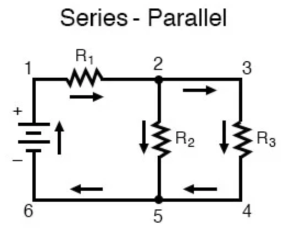In the circuit above, the battery has EMF of 12 V, R1 is 1 Ohm, R2 is 2 Ohms and R3 is 3 Ohms

Find the power dissipated by R3

Find the voltage drop across R1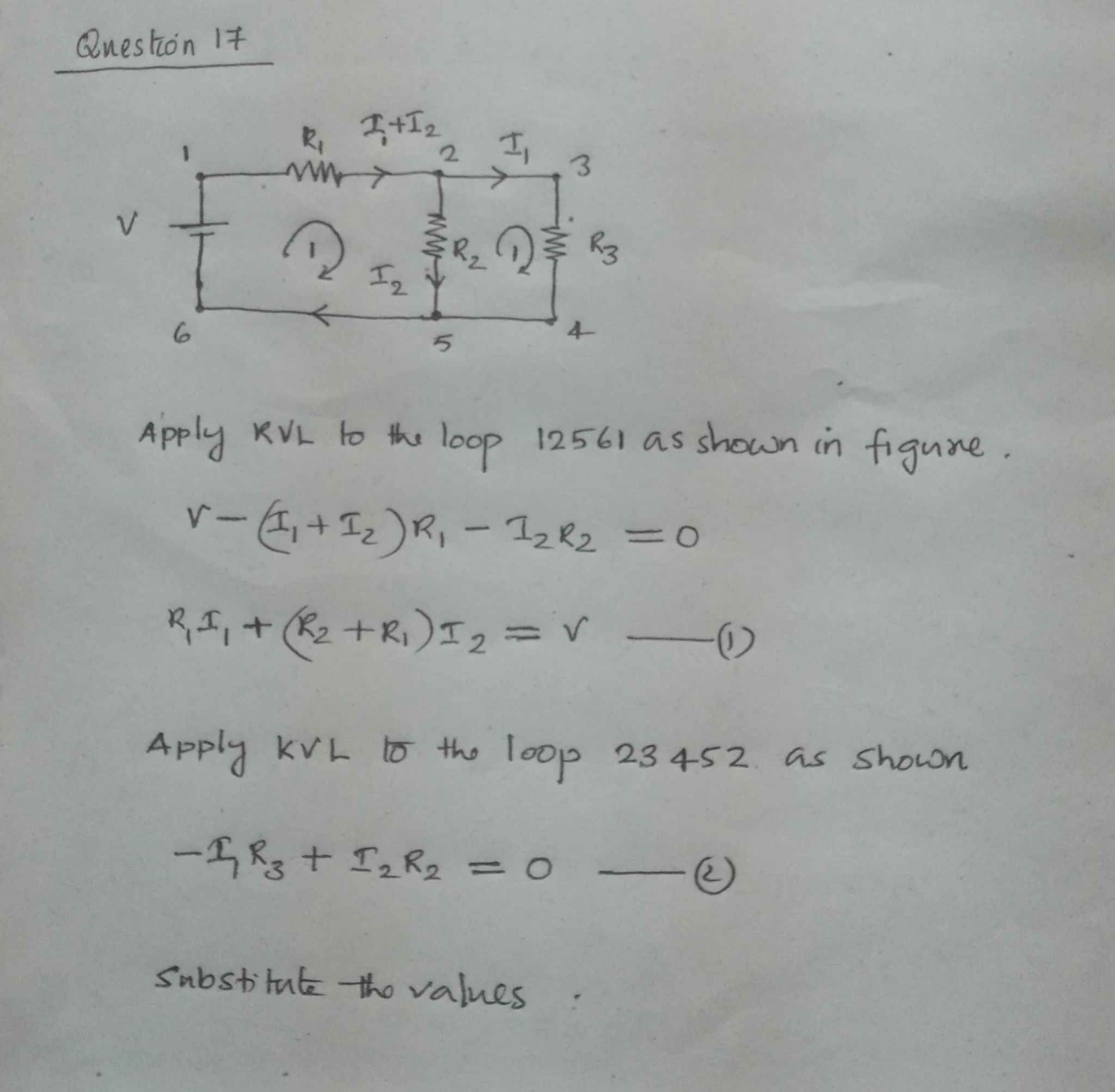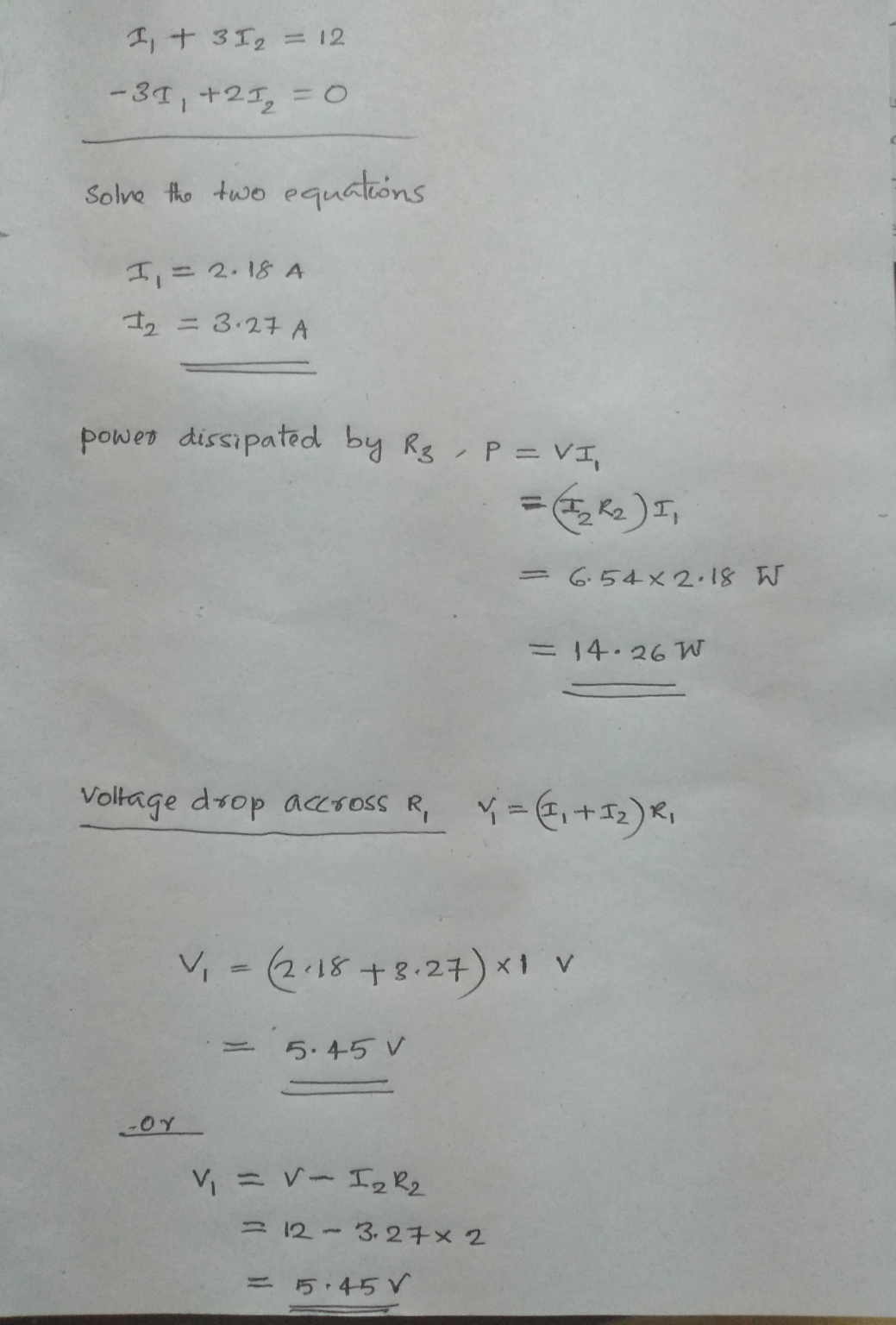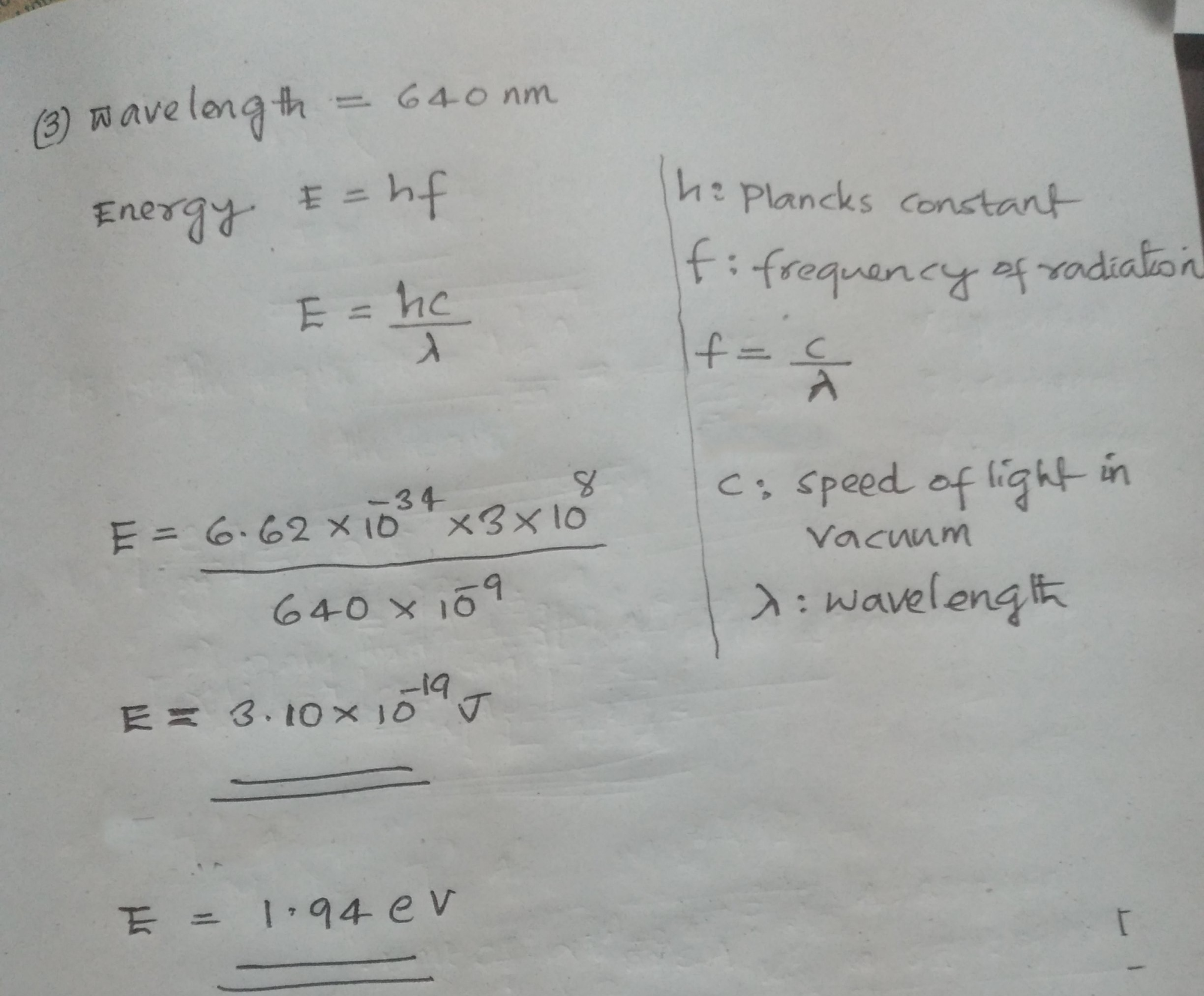#### Earn Coins

Coins can be redeemed for fabulous gifts.

Similar Homework Help Questions
• ### Consider the circuit below. R1 = 3 ohms, R2 = 6 ohms, R3 = 2 ohms,...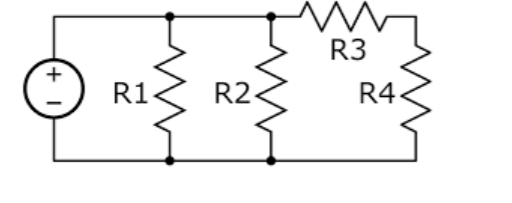Consider the circuit below. R1 = 3 ohms, R2 = 6 ohms, R3 = 2 ohms, R4 = 4 ohms, and the battery has a voltage of 12 V. Answer the following Pick the best answer for each True/False answer 1. R1 and R2 will both have a voltage across each of 12V. True or False. 2. R1 and R2 will both have the same current traveling through them. True or False. 3. R3 and R4 will both have a...

• ### For the following circuit, assume that R1 = 1 ohm, R2 = 2 ohms, R3 =...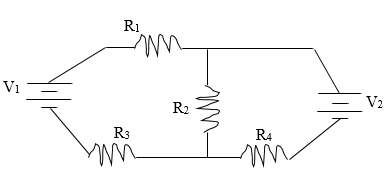For the following circuit, assume that R1 = 1 ohm, R2 = 2 ohms, R3 = 8 ohms, and R4 = 4 ohms. Also assume that V1 = 50 volts and V2 = 100 volts. Find the current through each of the resistors and the power dissipated through all of the resistors. (Hint: You’ll need to use Kirchoff’s Loop and Junction Rule) Vi R2 R3 R4 NV

• ### R1 12 R2 13 R3 V = 10 V, R1 = 50, R2 = 100, R3...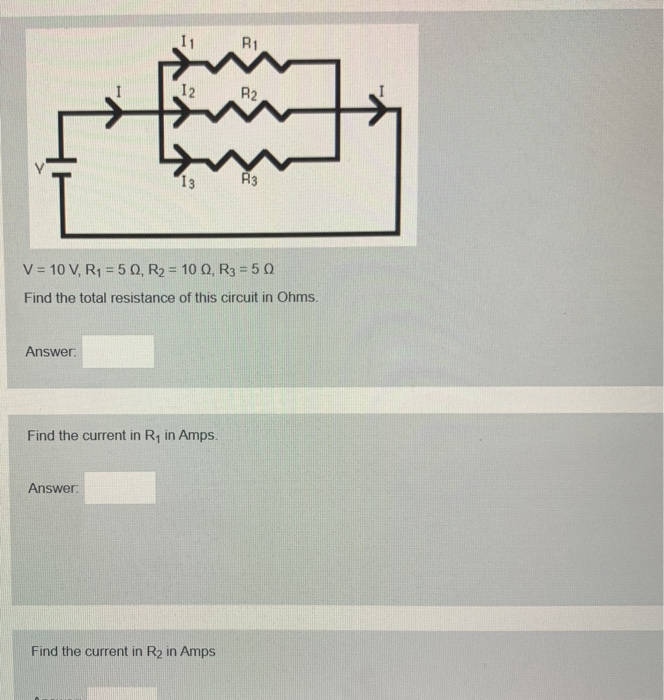R1 12 R2 13 R3 V = 10 V, R1 = 50, R2 = 100, R3 = 50 Find the total resistance of this circuit in Ohms. Answer: Find the current in Ry in Amps Answer: Find the current in R2 in Amps Find the current in R3 in Amps Answer: Find the voltage drop in Volts across R1. Answer: Find the voltage drop in Volts across R2. Answer:

• ### the voltage of the battery is 12V, and the values of the resistances are R1=5 ohms,...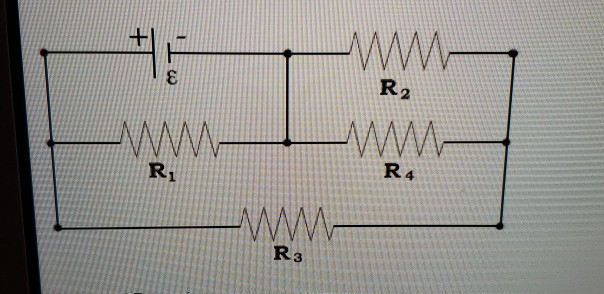the voltage of the battery is 12V, and the values of the resistances are R1=5 ohms, R2=10 ohms, R3= 15 ohms, and R4= 20 ohms. Answer the following questions: 1. R1 and R3 are in : 2. R2 and R4 are in: 3 Compare the magnitude of the current through R2 and R3. which is greater? 4. Compare the magnitude of the voltage across R1 and R3. which is greater? 5. Compare the magnitude of the voltage across R2 and...

• ### Consider a circuit with three resistors (R1 = 40.5 ohms, R2 = 54.8 ohms, and R3...

Consider a circuit with three resistors (R1 = 40.5 ohms, R2 = 54.8 ohms, and R3 = 30.8 ohms) connected to a 12.5 V battery as follows: R1 and R2 are connected in parallel. This combination is then connected in series to R3. Find the power dissipated in R1. Calculate the answer in watts (W) and rounded to three significant figures. please explain.

• ### for the circuit in Fig 10.3, V= 12V, R1= 4 ohms, R2=6ohms, and R3= 3 ohms....

for the circuit in Fig 10.3, V= 12V, R1= 4 ohms, R2=6ohms, and R3= 3 ohms. show that the power supplied by the battery (P=IV) is equal to that dissipated in the resistors (I^2R). what principle does this illustrate? Use the accompanying table. ( consider values significant to two decimal places.)

• ### In the circuit below given that R1 = 500 Ohm, R2 = 820 Ohm, R3 =...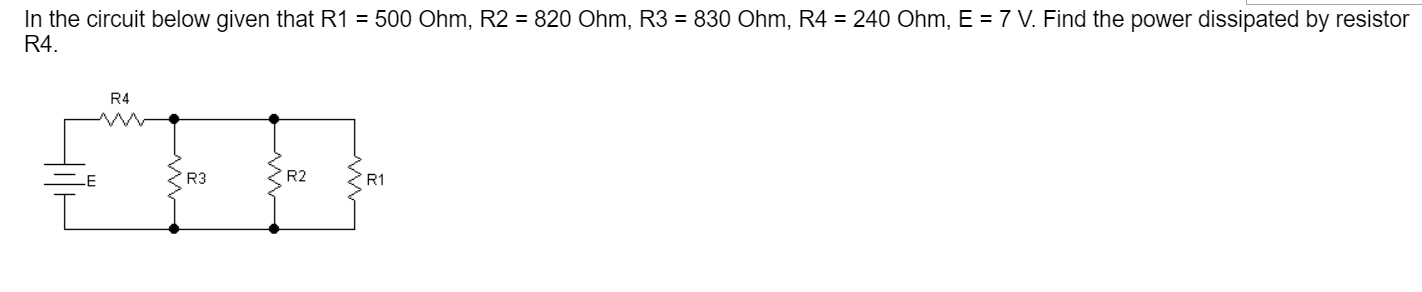In the circuit below given that R1 = 500 Ohm, R2 = 820 Ohm, R3 = 830 Ohm, R4 = 240 Ohm, E = 7 V. Find the power dissipated by resistor R4. R4 E R3 R2 R1

• ### 10. In the circuit below, the battery has an emf, ε, of 1.5 V and an...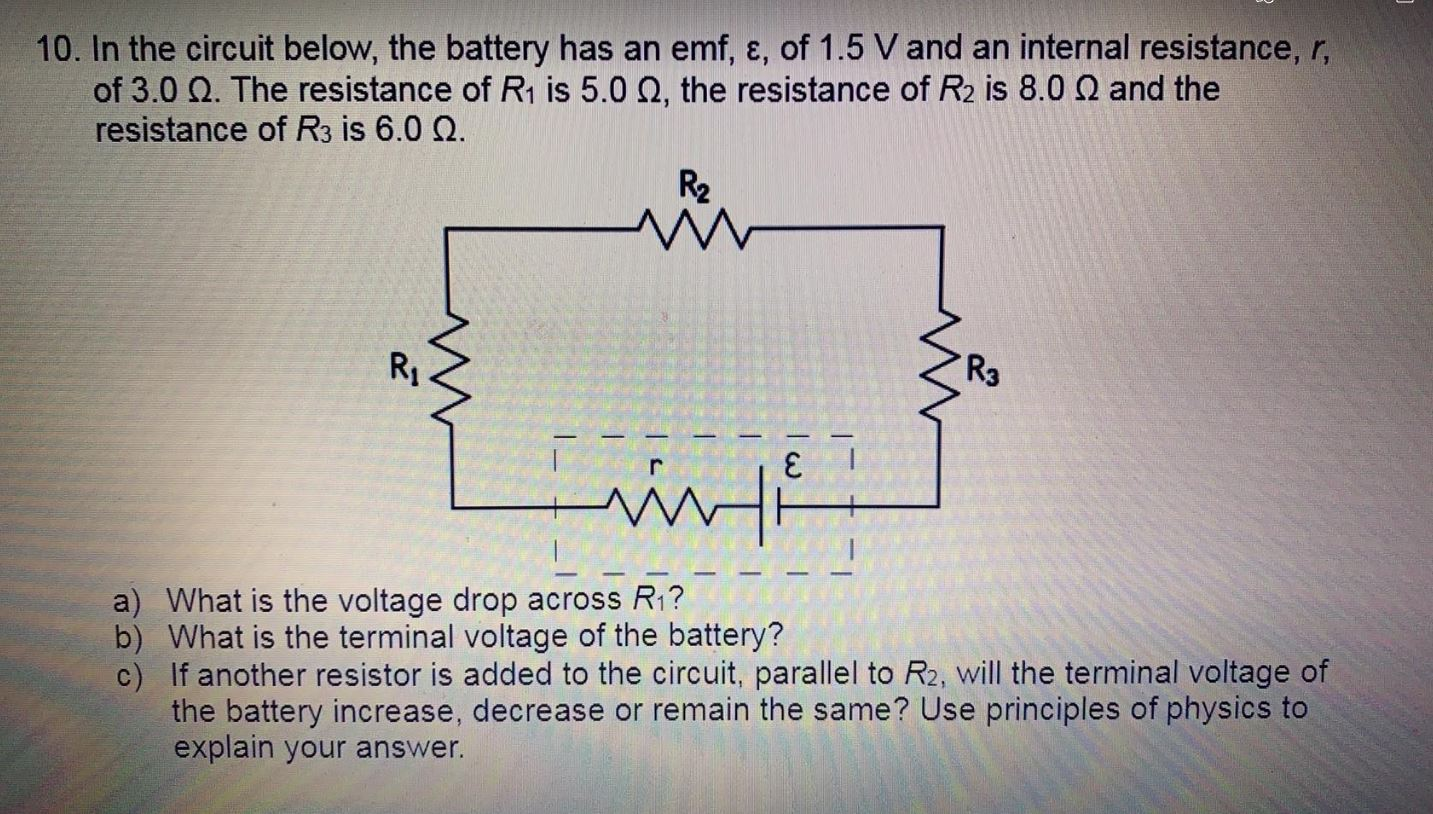10. In the circuit below, the battery has an emf, ε, of 1.5 V and an internal resistance, r, of 3.0 12. The resistance of R1 is 5.0 12, the resistance of R2 is 8.0 N and the resistance of R3 is 6.0 12. R2 w Ri R3 winti a) What is the voltage drop across R1? b) What is the terminal voltage of the battery? c) If another resistor is added to the circuit, parallel to R2, will the...

• ### Consider a circuit with four resistors in series. R1 R2 R3 R4 The battery has a...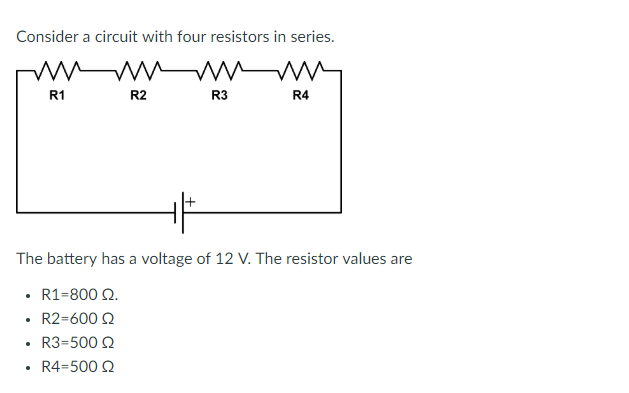Consider a circuit with four resistors in series. R1 R2 R3 R4 The battery has a voltage of 12 V. The resistor values are R1-800 Ω. R2-600 Ω R3-500 Q . R4-500Q Complete the following table by noting the voltage drop across each listed resistor. Resistor (Q) Voltage Drop (V) R1 00Select R3500Select ] 00 Select ] R2 R4

• ### Problem #1(20 points) Assuming R1 R2 = R3 = R4 = 10 ohm. Find 1. The...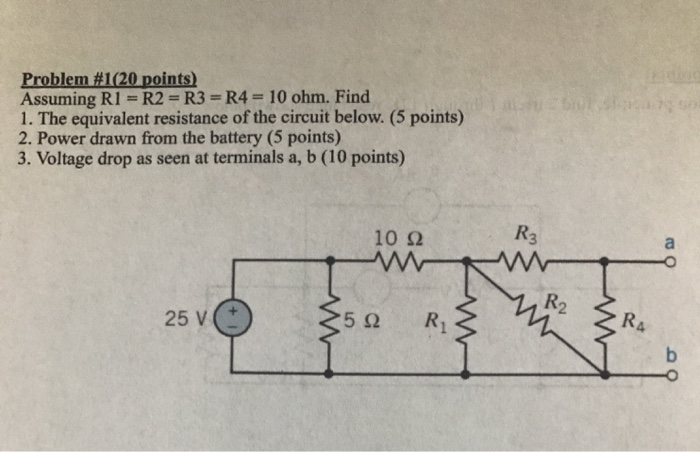Problem #1(20 points) Assuming R1 R2 = R3 = R4 = 10 ohm. Find 1. The equivalent resistance of the circuit below. (5 points) 2. Power drawn from the battery (5 points) 3. Voltage drop as seen at terminals a, b (10 points) R3 10 2 R2 RA R1 5Q 25 V b ww